﻿ Events you won't want to missLike   Tweet
 /* styles */ While the gala sold out quickly, there are still numerous ways to connect with the 2017 winners of the Dayton Literary Peace Prize over the next few weeks. Our public events are designed to reach out to readers who are interested in personal connections with DLPP writers and their books and we welcome you to become a part of the conversations about literature and peace. Additional information on even more connections can be found on our website under Outreach. Please join us in celebrating the power of the written word to promote peace.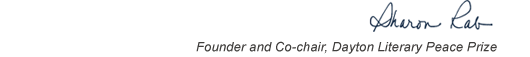table div table+table+table+table div table{width:100%;padding:0}table div table+table+table+table div table img{width:96.23%;padding:0;float:none}table div table+table+table+table div table td{width:100%;padding:0 1.88% 18px}/* styles */# Community Conversations: Books of the Dayton Literary Peace Prize

 table div table+table+table+table+table+table div table{width:100%;padding:0}table div table+table+table+table+table+table div table img{width:96.23%;padding:0;float:none}table div table+table+table+table+table+table div table td{width:100%;padding:0 1.88% 18px}/* styles *//* styles */ Join area scholars and community readers at the Dayton Metro Library for a conversation about books written by Colm Toíbín. Professors from area universities will be joined by a local writer to talk about his impact and artistry, essential themes, and the power of the written word to promote peace. If you’ve already read Colm Toíbín, or are just thinking about doing so, this conversation will enrich your appreciation and understanding of him and his work. This conversation is free and open to the public.

## Thursday, November 2, 2017 6:30 pm - 8:00 pm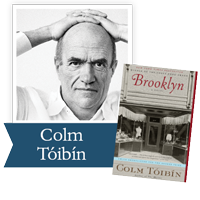Wright State department of English in partnership with the Dayton Public Library and the Dayton Literary Peace Prize

Dayton Public Library Main, The Eichelberger Forum, 1st Floor, Rm 103

Carol Loranger, Ph.D., Associate Professor and Chair of English, Wright State University, leads discussion about Colm Toíbín, the 2017 Richard C. Holbrooke Distinguished Achievement Award recipient. Community leader/readers include Sharon Short, Executive Director of the Antioch Writers’ Workshop and author of My One Square Inch of Alaska and Greg Anderson, Associate Professor of History at The Ohio State University and author of The Athenian Experiment: Building an Imagined Political Community in Ancient Attica, 508-490 B.C.

 table div table+table+table+table+table+table+table+table+table+table div table{width:100%;padding:0}table div table+table+table+table+table+table+table+table+table+table div table img{width:96.23%;padding:0;float:none}table div table+table+table+table+table+table+table+table+table+table div table td{width:100%;padding:0 1.88% 18px}/* styles */# Sunday Morning Conversation with the 2017 Dayton Literary Peace Prize Winners

 table div table+table+table+table+table+table+table+table+table+table+table+table div table{width:100%;padding:0}table div table+table+table+table+table+table+table+table+table+table+table+table div table img{width:96.23%;padding:0;float:none}table div table+table+table+table+table+table+table+table+table+table+table+table div table td{width:100%;padding:0 1.88% 18px}/* styles */## *Please note Daylight Savings Time ends Saturday at midnight.

 /* styles */ Sinclair Community College, Bldg 12—Ponitz Center (North End) Free Underground Parking—Bldg 12 (W. 4th Street Entrance) Please join us for a discussion of peace and literature with the current and former winners of the Dayton Literary Peace Prize. Writer, composer, editor, Christopher Cerf, former DLPP judge and current DLPP Honorary Advisory Board member, will moderate the panel made up of the 2017 winners of the Dayton Literary Peace Prize: Colm Tóibín, recipient of the Richard C. Holbrooke Distinguished Achievement Award; Patricia Engel, Fiction Winner for The Veins of the Ocean; David Wood, Nonfiction Winner for What Have We Done. Joining them on the panel will be previous winners: Viet Thanh Nguyen, 2016 Fiction Winner for The Sympathizer; Susan Southard, 2016 Nonfiction Winner for Nagasaki; James Hannaham, 2016 Fiction Runner-up for Delicious Foods; Gilbert King, 2013 Nonfiction Runner-up for Devil in the Grove (Gilbert is also a member of the DLPP Honorary Advisory Board); Andrew Krivák, 2012 Fiction Winner for The Sojourn. Reservations are required. RSVP to Linda Harrison by Tuesday, October 31. Cost is \$15.00 in cash or check payable to DLPP Foundation. There is limited seating. Participants will sign books after the program. Books will be available for purchase.
 table div table+table+table+table+table+table+table+table+table+table+table+table+table+table+table div table{width:100%;padding:0}table div table+table+table+table+table+table+table+table+table+table+table+table+table+table+table div table img{width:96.23%;padding:0;float:none}table div table+table+table+table+table+table+table+table+table+table+table+table+table+table+table div table td{width:100%;padding:0 1.88% 18px}/* styles */# Brooklyn: An Evening with Colm Tóibín

 table div table+table+table+table+table+table+table+table+table+table+table+table+table+table+table+table+table div table{width:100%;padding:0}table div table+table+table+table+table+table+table+table+table+table+table+table+table+table+table+table+table div table img{width:96.23%;padding:0;float:none}table div table+table+table+table+table+table+table+table+table+table+table+table+table+table+table+table+table div table td{width:100%;padding:0 1.88% 18px}/* styles */## Monday, November 6, 2017 7:30 pm at the Neon Movies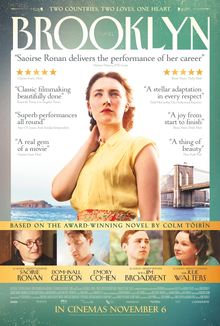The Dayton Literary Peace Prize and the 2017 Holbrooke Winner, Colm Tóibín, will proudly host a showing of Brooklyn on Monday, November 6, 2017, at THE NEON. The film, based on the novel by Colm Tóibín, captures the journey of an Irish immigrant landing in 1950s Brooklyn, where she quickly falls into a romance with a local. When her past catches up with her, however, she must choose between two countries and the lives that exist within.

Following the movie, Colm Tóibín will lead a discussion about the film adaptation of the novel with the audience. The showing begins at 7:30 pm, and tickets are available for \$25. Only 133 seats available. Purchase tickets here or at THE NEON. Tickets purchased through the link above will be available at the Will Call table located at the entrance of THE NEON.

## DLPP thanks the Miami Valley School, Digestive Specialists, Inc and Digestive Endoscopy Center LLC, and Square One Salon and Day Spa for their sponsorship of Brooklyn: An Evening with Author Colm Tóibín.

 table div table+table+table+table+table+table+table+table+table+table+table+table+table+table+table+table+table+table+table+table div table{width:100%;padding:0}table div table+table+table+table+table+table+table+table+table+table+table+table+table+table+table+table+table+table+table+table div table img{width:96.23%;padding:0;float:none}table div table+table+table+table+table+table+table+table+table+table+table+table+table+table+table+table+table+table+table+table div table td{width:100%;padding:0 1.88% 18px}/* styles */# Classical Tragedy and the Modern Imagination: A Conversation with Colm Tóibín

 table div table+table+table+table+table+table+table+table+table+table+table+table+table+table+table+table+table+table+table+table+table+table div table{width:100%;padding:0}table div table+table+table+table+table+table+table+table+table+table+table+table+table+table+table+table+table+table+table+table+table+table div table img{width:96.23%;padding:0;float:none}table div table+table+table+table+table+table+table+table+table+table+table+table+table+table+table+table+table+table+table+table+table+table div table td{width:100%;padding:0 1.88% 18px}/* styles */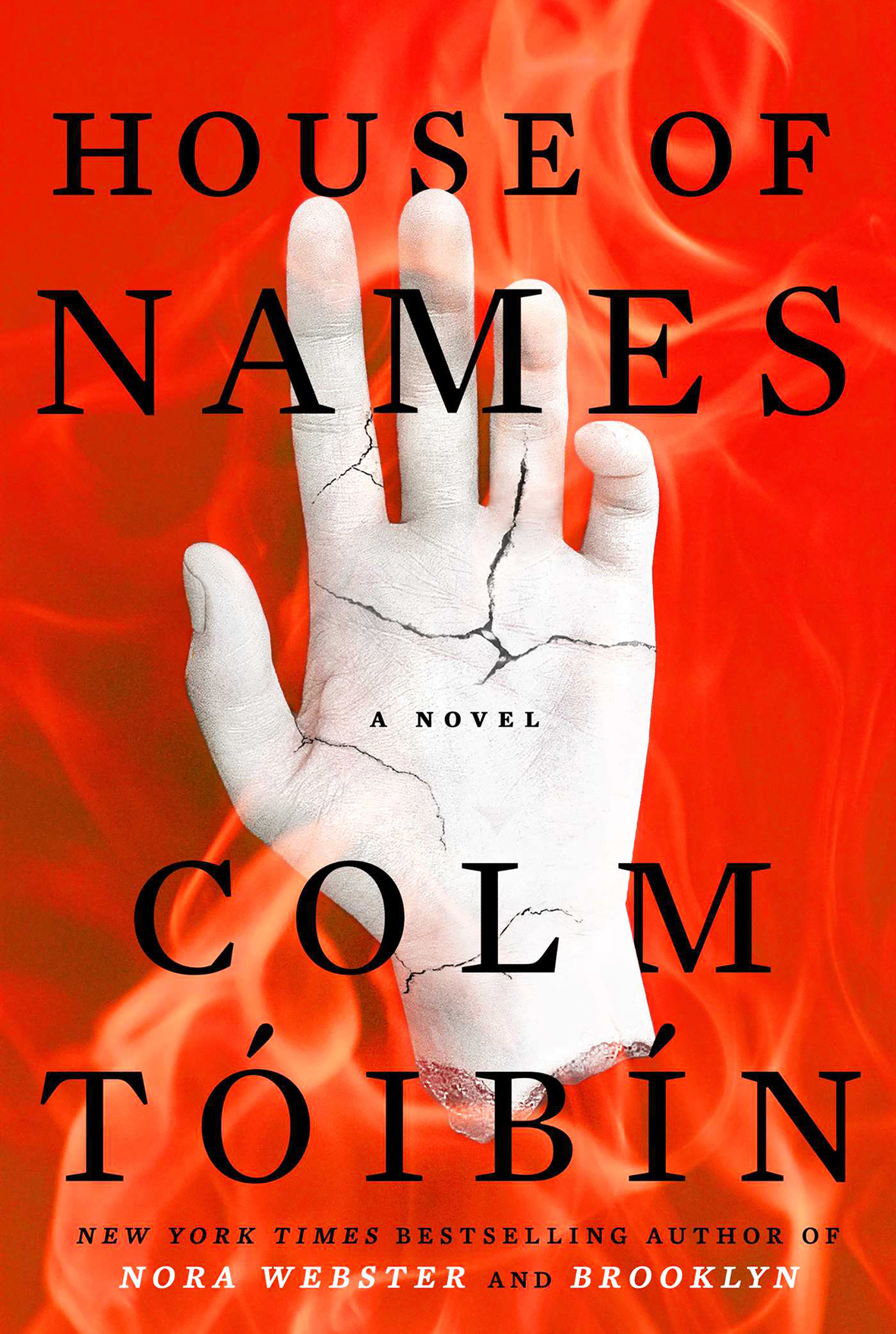## Monday, November 6th, 2017 2:00 pm – 3:00 pm

Wright State University Creative Arts Center, Stein Galleries

Join the 2017 Dayton Literary Peace Prize Holbrooke Distinguished Achievement Award winner, Colm Tóibín for a panel discussion at Wright State University. The discussion will focus on the use of classical tragedy in Tóibín’s novel, House of Names. This event is free and open to the campus community and the public.

 table div table+table+table+table+table+table+table+table+table+table+table+table+table+table+table+table+table+table+table+table+table+table+table+table div table{width:100%;padding:0}table div table+table+table+table+table+table+table+table+table+table+table+table+table+table+table+table+table+table+table+table+table+table+table+table div table img{width:96.23%;padding:0;float:none}table div table+table+table+table+table+table+table+table+table+table+table+table+table+table+table+table+table+table+table+table+table+table+table+table div table td{width:100%;padding:0 1.88% 18px}/* styles */# Conversations with David Wood, Nonfiction Winner

 table div table+table+table+table+table+table+table+table+table+table+table+table+table+table+table+table+table+table+table+table+table+table+table+table+table+table div table{width:100%;padding:0}table div table+table+table+table+table+table+table+table+table+table+table+table+table+table+table+table+table+table+table+table+table+table+table+table+table+table div table img{width:96.23%;padding:0;float:none}table div table+table+table+table+table+table+table+table+table+table+table+table+table+table+table+table+table+table+table+table+table+table+table+table+table+table div table td{width:100%;padding:0 1.88% 18px}/* styles */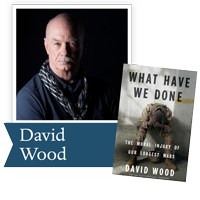## 10:30 am - 11:30 am

Edison State Community College,
Robinson Theater, 1973 Edison Dr, Piqua, OH

During his visit, author David Wood will discuss his award-winning novel, What Have We Done: The Moral Injury of Our Longest Wars, which chronicles the moral costs of the wars in Iraq and Afghanistan. Sharing numerous stories from individuals who served, Wood sheds light on the harrowing effect modern warfare has created on both a personal and national level. This event is offered free of charge and is open to the community. More information.

## 12:30 pm – 1:30 pm

Sinclair Community College, Library, 444 W Third St, Dayton, OH

A veteran war reporter, David Wood will discuss his award-winning book, What Have We Done: The Moral Injury of Our Longest Wars, which chronicles the moral costs of the wars in Iraq and Afghanistan. He is a staff correspondent for the Huffington Post, where he won the 2012 Pulitzer Prize for national reporting on severely wounded warriors. More information.

 table div table+table+table+table+table+table+table+table+table+table+table+table+table+table+table+table+table+table+table+table+table+table+table+table+table+table+table+table+table div table{width:100%;padding:0}table div table+table+table+table+table+table+table+table+table+table+table+table+table+table+table+table+table+table+table+table+table+table+table+table+table+table+table+table+table div table img{width:96.23%;padding:0;float:none}table div table+table+table+table+table+table+table+table+table+table+table+table+table+table+table+table+table+table+table+table+table+table+table+table+table+table+table+table+table div table td{width:100%;padding:0 1.88% 18px}/* styles */# Blocking Out Catastrophe: Images of Nuclear Denial and Empowerment

 table div table+table+table+table+table+table+table+table+table+table+table+table+table+table+table+table+table+table+table+table+table+table+table+table+table+table+table+table+table+table+table div table{width:100%;padding:0}table div table+table+table+table+table+table+table+table+table+table+table+table+table+table+table+table+table+table+table+table+table+table+table+table+table+table+table+table+table+table+table div table img{width:96.23%;padding:0;float:none}table div table+table+table+table+table+table+table+table+table+table+table+table+table+table+table+table+table+table+table+table+table+table+table+table+table+table+table+table+table+table+table div table td{width:100%;padding:0 1.88% 18px}/* styles */## Monday, October 30, 2017 7:00 pm - 8:30 pm

Wright Memorial Public Library, 1776 Far Hills Ave, Oakwood, Ohio 45419

Join Nigel Young, notable peace scholar and editor-in-chief of The Oxford International Encyclopedia of Peace, for which he won a DLPP Education Award, for a discussion of the many ways our society denies the true nature of an all-out nuclear war. It's unthinkable, so we don't think about it, and that attitude is reflected in cultural images and political action that don't represent the depth of destruction a nuclear war would bring. Learn how peace and nonviolent movements are confronting this issue by reimagining art, photography and political action that empowers people and counters our tendency to gloss over potential atrocity.

 table div table+table+table+table+table+table+table+table+table+table+table+table+table+table+table+table+table+table+table+table+table+table+table+table+table+table+table+table+table+table+table+table+table div table{width:100%;padding:0}table div table+table+table+table+table+table+table+table+table+table+table+table+table+table+table+table+table+table+table+table+table+table+table+table+table+table+table+table+table+table+table+table+table div table img{width:96.23%;padding:0;float:none}table div table+table+table+table+table+table+table+table+table+table+table+table+table+table+table+table+table+table+table+table+table+table+table+table+table+table+table+table+table+table+table+table+table div table td{width:100%;padding:0 1.88% 18px}/* styles */# Spirit of ’64: Miami University and the Enduring Legacy of Freedom Summer

 table div table+table+table+table+table+table+table+table+table+table+table+table+table+table+table+table+table+table+table+table+table+table+table+table+table+table+table+table+table+table+table+table+table+table+table div table{width:100%;padding:0}table div table+table+table+table+table+table+table+table+table+table+table+table+table+table+table+table+table+table+table+table+table+table+table+table+table+table+table+table+table+table+table+table+table+table+table div table img{width:96.23%;padding:0;float:none}table div table+table+table+table+table+table+table+table+table+table+table+table+table+table+table+table+table+table+table+table+table+table+table+table+table+table+table+table+table+table+table+table+table+table+table div table td{width:100%;padding:0 1.88% 18px}/* styles */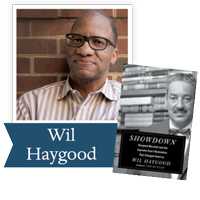## Saturday, Oct. 28, 2017 10:00 am - 11:30 am

Registration and refreshments begin at 9 a.m.
National Underground Railroad Freedom Center, 50 E. Freedom Way, Cincinnati, OH 45202

Miami University introduces Spirit of ’64: Miami University and the Enduring Legacy of Freedom Summer. This program will reflect on how Freedom Summer became the catalyst for progress and change in the United States, as well as how it permanently affected the fabric of Miami University.

Alumni Wil Haygood ’76 and Jeff Pegues ’92 will return to southwest Ohio for an event, open to the public, in which they each share their thoughts on race in America. Haygood's most recent book is Showdown: Thurgood Marshall and the Supreme Court Nomination That Changed America was a finalist for the 2016 Dayton Literary Peace Prize Nonfiction Award.

Cost is \$20 per person and includes light refreshments, lecture, museum entry and copies of Jeff and Wil’s most recent books. Registration has been extended. Space is limited.

Questions? Contact Michelle Rosecrans.

 table div table+table+table+table+table+table+table+table+table+table+table+table+table+table+table+table+table+table+table+table+table+table+table+table+table+table+table+table+table+table+table+table+table+table+table+table+table div table{width:100%;padding:0}table div table+table+table+table+table+table+table+table+table+table+table+table+table+table+table+table+table+table+table+table+table+table+table+table+table+table+table+table+table+table+table+table+table+table+table+table+table div table img{width:96.23%;padding:0;float:none}table div table+table+table+table+table+table+table+table+table+table+table+table+table+table+table+table+table+table+table+table+table+table+table+table+table+table+table+table+table+table+table+table+table+table+table+table+table div table td{width:100%;padding:0 1.88% 18px}/* styles */table div table+table+table+table+table+table+table+table+table+table+table+table+table+table+table+table+table+table+table+table+table+table+table+table+table+table+table+table+table+table+table+table+table+table+table+table+table+table+table div table{width:100%;padding:0}table div table+table+table+table+table+table+table+table+table+table+table+table+table+table+table+table+table+table+table+table+table+table+table+table+table+table+table+table+table+table+table+table+table+table+table+table+table+table+table div table img{width:96.23%;padding:0;float:none}table div table+table+table+table+table+table+table+table+table+table+table+table+table+table+table+table+table+table+table+table+table+table+table+table+table+table+table+table+table+table+table+table+table+table+table+table+table+table+table div table td{width:100%;padding:0 1.88% 18px}/* styles */